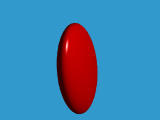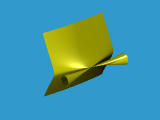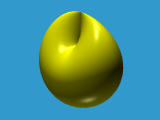# Algebraic SurfacesAlgebraic surfaces are surfaces whose points (x,y,z) satisfy a polynomial of three variables p(x,y,z)=0. For example, a sphere is an algebraic surface since it can be described as

(x - a)2 + (y - b)2 + (z - c)2 = r2
The degree of an algebraic surface is the highest degree of all of its terms. Thus, spheres are degree two algebraic surfaces. It is very difficult to display algebraic surfaces correctly because some of them may extend to infinity and some of them are extremely complicated.

POVRAY can raytrace any algebraic surface of degree less than or equal to 7. In general, this range is sufficient for most applications because algebraic surfaces of degree higher than 7 are rarely used and are very complex. POVRAY supports two forms. One for quadric, cubic and quartic surfaces which are commonly used. The other is a general form.```quadric {
< A, B, C >,
< D, E, F >,
< G, H, I >,
J
// pigment and finish
}
```
where these ten values are coefficients of a quadric polynomial as follows:
Ax2 + By2 + Cz2 + Dxy + Exz + Fyz + Gx + Hy + Iz + J = 0
For example, to trace the following quadric surface
10x2 + y2 + 2z2 + 3xy + 4xz + yz + 5x + y + 10z = 0
use the following
```quadric {
< 10,  1,  2 >,
<  3,  4,  1 >,
<  5,  1, 10 >,
0
// pigment, finish and transformations
}
```
Note that in general geometric transformations are required to bring the surface into your camera's coverage. Also note that the above equation is different from what has been discussed in class. The result a flat ellipsoid as shown below:### Cubic Surfaces

POVRAY uses the following cubic equation for tracing a cubic surface:
A1x3 + A2x2y + A3x2z + A4x2 + A5xy2 + A6xyz + A7xy + A8xz2 + A9xz + A10x + A11y3 + A12y2z + A13y2 + A14yz2 + A15yz + A16y + A17z3 + A18z2 + A19z + A20 = 0
There are 20 coefficients organized as follows:
```cubic {
< A1, A2, A3, ...., A20 >
sturm      // optional
// pigment, finish and transformations
}
```
All 20 coefficients are in a single vector. Note that a new keyword sturm is introduced. This keyword is used only when the degree of an algebraic surface is greater than 2. The purpose of using this keyword is for more accurate ray and object intersection. As a result, it is more time consuming than without using it. Charles Sturm was a well-known German mathematician of the last century who discovered a method for determining the ranges of the roots of a single-variable polynomial.

To trace the following cubic surface

x3 - 0.11111xz2 + y2 = 0
use the following:
```cubic {
//     x3  x2y  x2z  x2  xy2
< 1,  0,   0,   0,   0,
//     xyz xy   xz2       xz   x
0,  0,  -0.11111,  0,   0,
//     y3  y2z  y2  yz2  yz
0,  0,   1,  0,   0,
//     y   z3   z2  z    Const
0,  0,   0,  0,   0 >
sturm
clipped_by {
box { < -2, -2, -2 >, < 2, 2, 2 > }
}
}
```

Because cubic surfaces extend to infinity, frequently a clipped_by {} is used to cut off unwanted part. It may require several tries to get the result right. Here is the result:### Quartic Surfaces

Quartic surfaces require 35 coefficients as shown below:
A1x4 + A2x3y + A3x3z + A4x3 + A5x2y2 + A6x2yz + A7x2y + A8x2z2 + A9x2z + A10x2 + A11xy3 + A12xy2z + A13xy2 + A14xyz2 + A15xyz + A16xy + A17xz3 + A18xz2 + A19xz + A20x + A21y4 + A22y3z + A23y3 + A24y2z2 + A25y2z + A26y2 + A27yz3 + A28yz2 + A29yz + A30y + A31z4 + A32z3 + A33z2 + A34z + A35 = 0

These coefficients are organized as follows:

```quartic {
< A1, A2, ...., A35 >
sturm      // optional
// pigment, finish and transformations
}
```
To trace the following quartic surface
x4 + x2y2 - 3x2y + 2.5x2z2 + 1.5y2z2 - 3yz2 + 1.5z4 = 0
use the following
```quartic {
//     x^4   x^3y   x^3z   x^3   x^2y^2
< 1,    0,     0,     0,    1,
//     x^2yz x^2y   x^2z^2 x^2z  x^2
0,    -3,    2.5,     0,    0,
//     xy^3  xy^2z  xy^2   xyz^2 xyz
0,    0,     0,     0,    0,
//     xy    xz^3   xz^2   xz    x
0,    0,     0,     0,    0,
//     y^4   y^3z   y^3    y^2z^2 y^2z
0,    0,     0,     1.5,   0,
//     y^2   yz^3   yz^2   yz    y
0,    0,     -3,    0,   0
//     z^4   z^3    z^2    z     Const
1.5,    0,     0,     0,    0 >
sturm
// pigment, finish and transformations
}
```

The result is the following well-known cross-cup surface### General Algebraic Surfaces

For general algebraic surfaces, use the following syntax:
```poly {
n,                         // degree of the surface
< A1, A2, ..... >          // coefficients
sturm                      // optional
// pigment, finish and transformations
}
```
where the degree n can be an integer in the range 2 and 7. The correspondence between coefficients and their positions in the vector is quite tedious and will not be listed here. If you need it, please click here to download a PostScript format table.